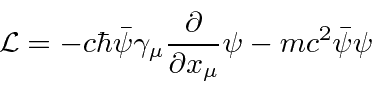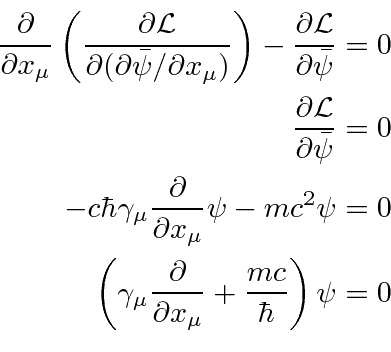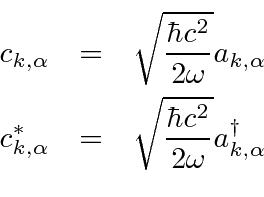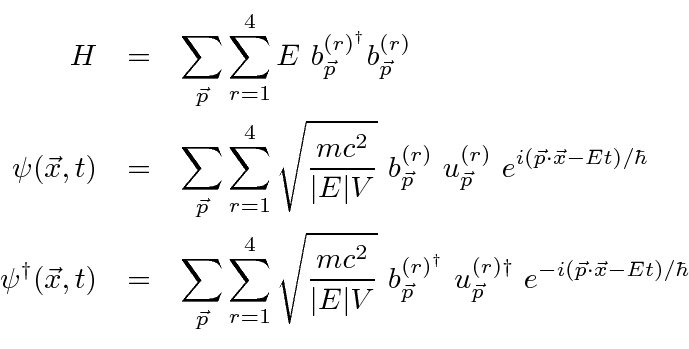## Quantization of the Dirac Field

The classical free field Lagrangian density for the Dirac electron field is.The independent fields are considered to be the 4 components ofand the four components of. This Lagrange density is a Lorentz scalar that depends only on the fields. The Euler-Lagrange equation using theindependent fields is simple since there is no derivative ofin the Lagrangian.This gives us the Dirac equation indicating that this Lagrangian is the right one. The Euler-Lagrange equation derived using the fieldsis the Dirac adjoint equation,again indicating that this is the correct Lagrangian if the Dirac equation is assumed to be correct.

To compute the Hamiltonian density, we start by finding the momenta conjugate to the fields.There is no time derivative ofso those momenta are zero. The Hamiltonian can then be computed.We may expand the fieldin the complete set of plane waves either using the four spinorsforor using the electron and positron spinorsandfor. For economy of notation, we choose the former with a plan to change to the later once the quantization is completed.The conjugate can also be written out.Writing the Hamiltonian in terms of these fields, the formula can be simplified as followswhere previous results from the Hamiltonian form of the Dirac equation and the normalization of the Dirac spinors have been used to simplify the formula greatly.

Compare this Hamiltonian to the one used to quantize the Electromagnetic fieldfor which the Fourier coefficients were replaced by operators as follows.The Hamiltonian written in terms of the creation and annihilation operators is.By analogy, we can skip the steps of making coordinates and momenta for the individual oscillators, and just replace the Fourier coefficients for the Dirac plane waves by operators.(Since the Fermi-Dirac operators will anti-commute, the analogy is imperfect.)

The creation an annihilation operatorsandsatisfy anticommutation relations.is the occupation number operator. The anti-commutation relations constrain the occupation number to be 1 or 0.

A state of the electrons in a system can be described by the occupation numbers (0 or 1 for each plane wave). The state can be generated by operation on the vacuum state with the appropriate set of creation operators.

Jim Branson 2013-04-22